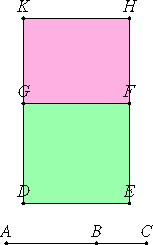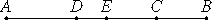# Proposition 41

If two straight lines incommensurable in square which make the sum of the squares on them medial and the rectangle contained by them medial and also incommensurable with the sum of the squares on them are added together, then the whole straight line is irrational; let it be called the side of the sum of two medial areas.

Let two straight lines AB and BC incommensurable in square and satisfying the given conditions be added together.

X.35

I say that AC is irrational.II.4

Set out a rational straight line DE. Apply to DE the rectangle DF equal to the sum of the squares on AB and BC, and apply to DE the rectangle GH equal to twice the rectangle AB by BC. Then the whole DH equals the square on AC.

X.22

Now, since the sum of the squares on AB and BC is medial, and equals DF, therefore DF is also medial. And it is applied to the rational straight line DE, therefore DG is rational and incommensurable in length with DE. For the same reason GK is also rational and incommensurable in length with GF, that is, DE.

Since the sum of the squares on AB and BC is incommensurable with twice the rectangle AB by BC, therefore DF is incommensurable with GH, so that DG is also incommensurable with GK.

X.36

And they are rational, therefore DG and GK are rational straight lines commensurable in square only. Therefore DK is irrational and what is called binomial.

X.Def.I.4

But DE is rational, therefore DH is irrational, and the side of the square which equals it is irrational.

But AC is the side of the square equal to HD, therefore AC is irrational. Let it be called the side of the sum two medial areas.

Q.E.D.

# Proposition 41

And that the aforesaid irrational straight lines are divided only in one way into the straight lines of which they are the sum and which produce the types in question we will now prove after premising the following lemma.

Set out the straight line AB, cut the whole into unequal parts at each of the points C and D, and let AC be supposed greater than DB.

I say that the sum of the squares on AC and CB is greater than the sum of the squares on AD and DB.

Bisect AB at E.

Since AC is greater than DB, subtract DC from each, therefore the remainder AD is greater than the remainder CB.But AE equals EB, therefore DE is less than EC. Therefore the points C and D are not equidistant from the point of bisection.

II.5

Since the rectangle AC by CB together with the square on EC equals the square on EB, and, further, the rectangle AD by DB together with the square on DE equals the square on EB, therefore the rectangle AC by CB together with the square on EC equals the rectangle AD by DB together with the square on DE.

And of these the square on DE is less than the square on EC, therefore the remainder, the rectangle AC by CB, is also less than the rectangle AD by DB so that twice the rectangle AC by CB is also less than twice the rectangle AD by DB.

Therefore the remainder, the sum of the squares on AC and CB, is greater than the sum of the squares on AD and DB.

Q.E.D.

## Guide

This proposition is used in X.59, X.65 and X.70.

The lemma is used in X.44.